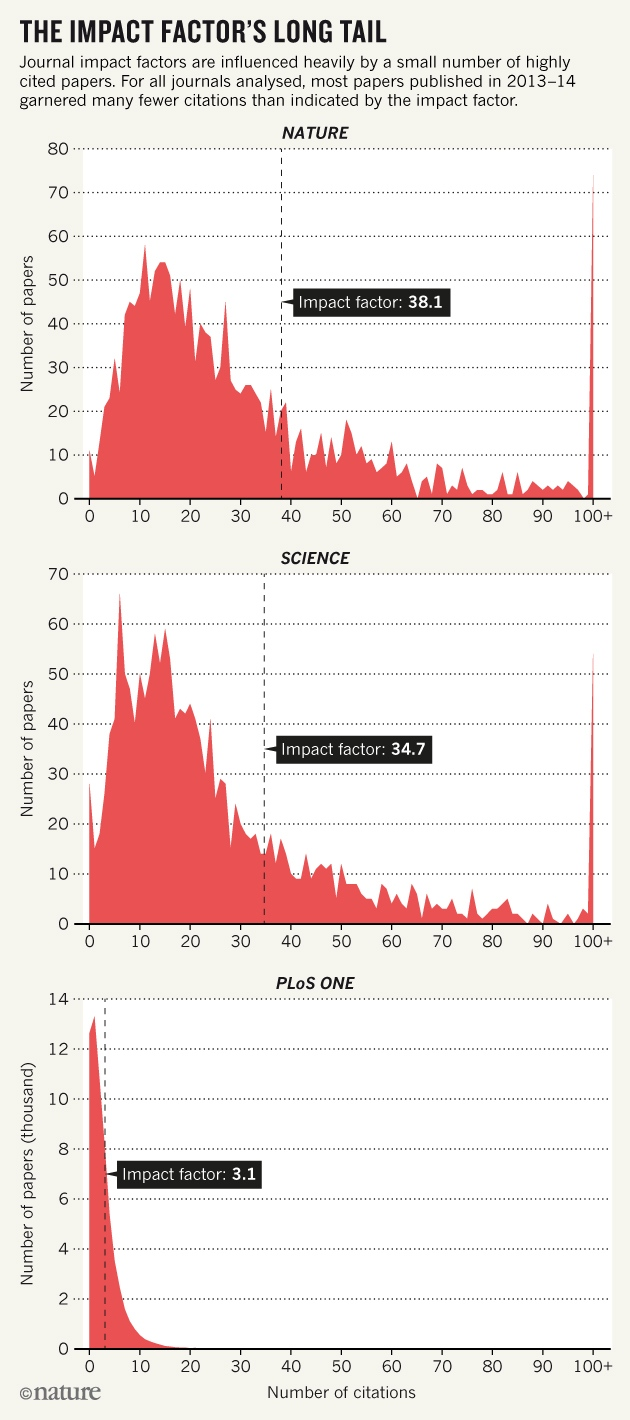## sampling the mean

Posted in Kids, R, Statistics with tags , , , , , on December 12, 2019 by xi'an

A challenge found on the board of the coffee room at CEREMADE, Université Paris Dauphine:

When sampling with replacement three numbers in {0,1,…,N}, what is the probability that their average is (at least) one of the three?

With a (code-golfed!) brute force solution of

```mean(!apply((a<-matrix(sample(0:n,3e6,rep=T),3)),2,mean)-apply(a,2,median))
```

producing a graph pretty close to 3N/2(N+1)² (which coincides with a back-of-the-envelope computation):## a discovery that mean can be impacted by extreme values

Posted in University life with tags , , , , , , on August 6, 2016 by xi'an

A surprising editorial in Nature about the misleading uses of impact factors, since as means they are heavily impacted by extreme values. With the realisation that the mean is not the median for skewed distributions…To be fair(er), Nature published a subsequent paper this week about publishing additional metrics like the two-year median.

## an ABC experiment

Posted in Books, pictures, R, Statistics, University life with tags , , , , , , , , on November 24, 2014 by xi'anIn a cross-validated forum exchange, I used the code below to illustrate the working of an ABC algorithm:

```#normal data with 100 observations
n=100
x=rnorm(n)
#observed summaries

#normal x gamma prior
priori=function(N){
return(cbind(rnorm(N,sd=10),
1/sqrt(rgamma(N,shape=2,scale=5))))
}

ABC=function(N,alpha=.05){

prior=priori(N) #reference table

#pseudo-data
summ=matrix(0,N,2)
for (i in 1:N){
xi=rnorm(n)*prior[i,2]+prior[i,1]
}

#normalisation factor for the distanceAfter thinking about this for a while, I went back to my workbench to check the difference with using mean and variance. To my greater surprise, I found hardly any difference! Using the almost exact ABC with 10⁶ simulations and a 5% subsampling rate returns exactly the same outcome. (The first row above is for the sufficient statistics (mean,standard deviation) while the second row is for the (median,mad) pair.) Playing with the distance does not help. The genuine posterior output is quite different, as exposed on the last row of the above, using a basic Gibbs sampler since the posterior is not truly conjugate.